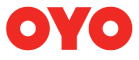New update is available. Click here to update.
Topics

# Search In A Row Wise And Column Wise Sorted Matrix

Moderate0/80
Average time to solve is 15m+34 more companies

## Problem statement

You are given an 'N * N' matrix of integers where each row and each column is sorted in increasing order. You are given a target integer 'X'.

Find the position of 'X' in the matrix. If it exists then return the pair {i, j} where 'i' represents the row and 'j' represents the column of the array, otherwise return {-1,-1}

For example:
``````If the given matrix is:
[ [1, 2, 5],
[3, 4, 9],
[6, 7, 10]]
We have to find the position of 4. We will return {1,1} since A = 4.
``````
Detailed explanation ( Input/output format, Notes, Images )
Sample Input 1:
``````2
3 4
1 2 5
3 4 9
6 7 10
2 5
4 5
8 6
``````
Sample Output 1:
``````1 1
0 1
``````
Constraints:
``````1 ≤ T ≤ 10
1 ≤ N ≤ 10^3
1 ≤ X ≤ 10^6
1 ≤ Aij ≤ 10^6

where 'T' is the number of test cases, 'N' is the number of rows and columns, 'X' is the target value, and Aij is the elements of the matrix.
Time Limit : 1 sec
``````
Explanation of Input 1:
``````The first test case is already explained in the problem statement.
The second test case,  the given matrix is:
[[4, 5],
[5, 6]]
We have to find the position of 5. So we return {0,1}.
``````
Sample Input 2:
``````2
3 16
2 4 8
3 6 9
4 7 16
1 10
4
``````
Sample Output 2
``````2 2
-1 -1
``````Console